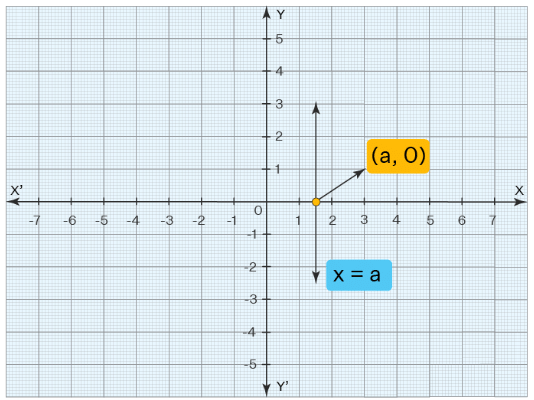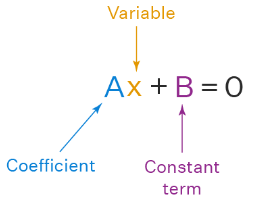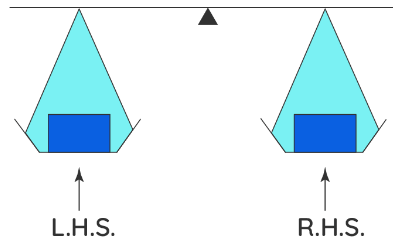Linear Equations in One Variable

# Linear Equations in One Variable - The Complete SAT Course - Class 10

Linear equation in one variable is the basic equation used to represent and solve for an unknown quantity. It can be easily represented graphically and it is always a straight line. The linear equation is an easy way of representing a math statement. Any variable or symbol can be used to represent unknown quantities but generally, a variable 'x' is used to represent the unknown quantity in the linear equation in one variable. Solving a linear equation includes a set of simple methods. The variables are isolated on one side of the equation and the constants are isolated to another side of the equation, to obtain the final value of the unknown quantity.

### What is Linear Equation in One Variable?

Before learning about linear equation in one variable, let us quickly go through the meaning of linear equations. A linear equation is a type of equation in which the degree of each variable in the equation is exactly equal to one. Linear equations in one variable are those equations in which there is only one variable present, and there is only one solution of the equation. When it is drawn on a graph, it appears to be a straight line either horizontally or vertically.A linear equation in one variable is of the form of ax+b=0, where a and b are any two integers and x is an unknown variable having only one solution. Let us understand this by taking an example- "4 added to a certain number gives 10". Find that number. How can we represent this problem in a simpler way? We can say, x + 4 = 10 , find x. We assigned a variable to that number; this is called an equation. It helps us write large problems like this in a shorter way. This equation has one variable which is x and the highest power of x is one. These kinds of equations are known as linear equations in one variable because the degree of the variable x is one.

### Solving Linear Equation in One Variable

The general form of a linear equation in one variable is Ax + B = 0. Here A is the coefficient of x, x is the variable, and B is the constant term. The coefficient and the constant term should be segregated to find the final solution of this linear equation.Now, let us look at how to solve a one-variable linear equation. An equation is like a weighing balance with equal weights on both sides.If we add or subtract the same number from both sides of an equation, it still holds. Similarly, if we multiply or divide the same number into both sides of an equation, it still holds. Consider the equation, 3x-2=4. We will perform mathematical operations on the LHS and the RHS so that the balance is not disturbed. Let's add 2 on both sides to reduce the LHS to 3x. This will not disturb the balance. The new LHS is 3x-2+2=3x and the new RHS is 4+2=6. Now let's divide both sides by 3 to reduce the LHS to x. Thus, we have 3x/3=6/3. Hence we have x = 2.
The above steps to solve linear equations in one variable can be summarized in the below-listed points.

• Step-1: Keep the variable term on one side and constants on another side of the equation by adding or subtracting on both sides of the equation.
• Step-2: Simplify the constant terms.
• Step-3: Isolate the variable on one side by multiply or dividing it into both sides of the equation.
• Step-4: Simplify and write the answer.

Question for Linear Equations in One Variable
Try yourself:What is the general form of a linear equation in one variable?

### Linear Equation in One Variable vs Non-Linear Equations

Apart from linear equations in one variable, we have other non-linear equations, which have numerous applications in geometry, trigonometry, and calculus. Linear equations in one variable are single-degree equations and are represented as a line on a coordinate plane. A non-linear equation on the other hand is a curve or non-linear representation on the coordinate axis. A nonlinear equation is of a higher degree. Few examples of non-linear equations are the equation of curves such as a circle, parabola, ellipse, hyperbola.
Some of the samples of linear equations are x = 5, 3x + 7 = 9, 4x + 2y = 11. And some of the examples of non-linear equations are the equation of a circle - x2 + y2 = 25, equation of a elipse - x2/9 + y2/16 = 1, equation of a hyperbola - x2 /16 - y2/ 25 = 1.

Important Notes
The following points help us in clearly summarizing the concepts involved in linear equations in one variable.

• The degree of the variable in linear equations should be exactly equal to one.
• The graph of a linear equation in one variable is a straight line, either horizontal or vertical.
• The solution of a linear equation in one variable is unaffected if any number is added, subtracted, multiplied, or divided on both sides of the equation.

### Linear Equation in One Variable Examples

Example 1: Twenty years ago, Mikkel's age was one-third of what it is now. What is Mikkel's present age?
Solution: We can write the given information by using a linear equation in one variable. Let Mikkel's present age be x years. Twenty years ago, Mikkel's age was (x-20) years. According to the given information, x - 20= x/3
3(x - 20)= x
3x - 60= x
3x - x= 60
2x= 60
x= 60/2
x= 30
Therefore the present age of Mikkel is 30 years.

Example 2: David worked as a stenographer. In June, he was paid \$50 per day. However, \$10 per day was deducted for the days he remained absent. He received \$900 for the number of days he worked. How many days did he work?
Solution: Let the number of days he worked be x days. Hence, the number of days he did not work will be = 30 - x. He was paid \$50 for each day he worked and \$10 was deducted for each day he did not work. At the end of the month, he received \$900. According to the given information, we can form a linear equation in one variable as, 50(x) - 10(30 - x) = 900
50x - 300 + 10x = 900
60x = 900 + 300
x = 1200/60
x= 20
Therefore David worked for 20 days.

Example 3: Find the perimeter of the square whose side length x units is given in the form of an equation as 2x/3- 5/6 = 0
Solution: Given, the side-length of the square is 2x/3- 5/6=0. It is in the form of a linear equation in one variable which is x. First, we need to solve this equation to find the value of x.
2x/3- 5/6=0
2x/3- 5/6 + 5/6=0+ 5/6 (adding 5/6 on both the sides)
2x/3= 5/6
x = 5/6 × 3/2
x = 5/4 units
Hence, the side length of the square is 5/4 units. Now, to find the perimeter of the square, we need to multiply the side length by 4.
The perimeter of a square = 5/4 × 4=5 units.
Therefore the perimeter of the square is 5 units.

The document Linear Equations in One Variable | The Complete SAT Course - Class 10 is a part of the Class 10 Course The Complete SAT Course.
All you need of Class 10 at this link: Class 10

## The Complete SAT Course

445 videos|216 docs|171 tests

## The Complete SAT Course

445 videos|216 docs|171 tests
Signup to see your scores go up within 7 days! Learn & Practice with 1000+ FREE Notes, Videos & Tests.
10M+ students study on EduRev
Track your progress, build streaks, highlight & save important lessons and more!(Scan QR code)
Related Searches

,

,

,

,

,

,

,

,

,

,

,

,

,

,

,

,

,

,

,

,

,

;